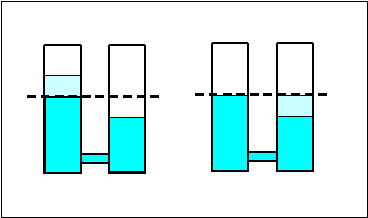Fluids at rest: two connected cylinders

Homework Statement

[This probably seems really easy to the physics whizzes out there but...*blushes*]

Two identical cylindrical vessels with their bases at the same level each contain a liquid of density 1.30x103 kg/m3. The area of each base is 4.00cm2, but in one vessel the liquid height is 0.854 m and in the other it is 1.560 m. Find the work done by the gravitational force in equalising the levels when the two vessels are connected.

Homework Equations

p2=p0 + $$\rho$$gh

p=F/A
$$\rho$$=m/V
p=$$\rho$$gh

The Attempt at a Solution

Well, it seems that this question has gone beyond the knowledge imparted earlier in the chapter this is in - either that or I have a lack of grey matter =]. However, I think I'm nearly there. The solution is as follows.

Find the pressures in the first and second cylinders.

p1= 1.3x103 x 9.8m/s2 x 0.854 m = 10,880 Pa

p2= 1.3x103 x 9.8m/s2 x 1.56 m = 19,874 Pa

$$\Delta$$p = 8,994 Pa

F= p x A = 8,994 Pa x 4x10-4 m2 = 3.60 N

Well, I've found the force due to the pressure difference. I'm not quite sure whether that is the correct approach though.

Now the work done by the gravitational force must be found. This is where I'm stuck. Is it as simple as multiplying the force - calculated above - by the change in height of the water? Or should I use U=-GMm/r?

I calculated the height of the equalised water to be 0.7 m. I did this by finding the height at which it would have to be for the water to cause a pressure of 8,994Pa.

EDIT: I suspect the height I have calculated is wrong.

Last edited:

Redbelly98
Staff Emeritus
Homework Helper
Welcome to PFI calculated the height of the equalised water to be 0.7 m. I did this by finding the height at which it would have to be for the water to cause a pressure of 8,994Pa.

EDIT: I suspect the height I have calculated is wrong.

Well, the problem with that height is that it's lower than both starting heights were to begin with.

Given the two starting heights, and the fact that the base areas are the same, what do you think the final equilibrium height should be? Hint: You won't need to calculate any forces for this part.

Hopefully I've thought along the right lines...

The maximum height of water one cylinder could contain is 1.560 m + 0.854 m = 2.414 m. If both cylinders are to equalise, they must contain the same mass, and hence volume, of water. This requires them to have the same height of water which is 2.414/2 m = 1.207 m.

Redbelly98
Staff Emeritus
Homework Helper
Yes, good.

Now, changing from the original two heights to the final height involves taking a certain volume of liquid, and lowering it by a certain amount.

If you can figure that part out (the volume of liquid that moved, and the change in height for that volume), next you would find the corresponding change in potential energy. And that is the work done by gravity.

hmm... (1.207 - .854) = .353
(1.207 - 1.560) = -.353

For one of the columns, gravity would do positive work, and for the other, negative work. And since the magnitude of the work is the same, the total work would just equal zero... or did I miss something?...

hmm... (1.207 - .854) = .353
(1.207 - 1.560) = -.353

For one of the columns, gravity would do positive work, and for the other, negative work. And since the magnitude of the work is the same, the total work would just equal zero... or did I miss something?...

That's what I was thinking. I'll have an attempt at Redbelly98's suggested solution and see if that leads to your answer.

Redbelly98
Staff Emeritus